• ## 变换矩阵

千次阅读 2018-10-27 16:58:25
1、变换矩阵 变换矩阵可以分解为缩放，旋转，平移矩阵的乘积： M = T * R * S - 右手坐标系 当均匀缩放时，旋转和缩放可以交换顺序 缩放和平移不可以交换顺序 2、子坐标系与父坐标系 由在父坐标系中的坐标位置...
1、变换矩阵
变换矩阵可以分解为缩放，旋转，平移矩阵的乘积：
M = T * R * S - 右手坐标系
当均匀缩放时，旋转和缩放可以交换顺序
缩放和平移不可以交换顺序
2、子坐标系与父坐标系
由在父坐标系中的坐标位置P，和三根轴X，Y，Z可定义一个子标系，按列构成一个变换矩阵[X,Y,Z,P]，这个矩阵构成由子标系变换到父坐标系的变换矩阵。DX里面需要转置一下。即按行构造。
一个例子是视图矩阵的构建，子空间由视点和三根正交轴定义，视图矩阵则是由它们构成的列矩阵的仿射求逆-由世界变换到视图空间。
另一个例子世界变换矩阵，它由新模型坐标系的位置和三根轴【定义在世界空间】按列构成。即由模型空间变换到世界空间。初始情况模型空间与世界空间是对齐的。
3、投影矩阵-OpenGL
透视投影矩阵：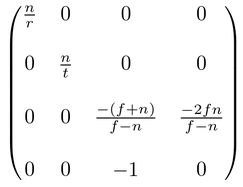可以知道投影变换后的w=-Ze，即在视图空间的深度
在投影面上的坐标Xp, Yp为：Xp=n*Xe/-Ze=Xclip/w*r，Yp=n*Ye/-Ze=Yclip/w*t
投影后Ze从[-n,-f]线性映射到[-n,f]，除以w后进一步非线性映射到[-1,1]
透视投影除以w后，视景体的八个角点【视图空间坐标】分别映射到NDC空间中的八个角点，如[r,t,-n,1]映射到[1,1,-1,1]，[f*r/n,f*t/n,-f,1]映射到[1,1,1,1]
正交投影矩阵：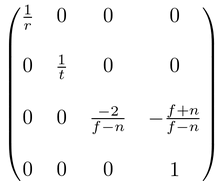变换后w=1，Xe，Ye，Ze被线性映射到-1~1，而透视投影是非线性映射，因而有Z-Fighting问题。
4.应用例子：从深度图重建位置
延迟渲染，SSR等特效都要求由深度信息重建位置信息，如果深度是归一化过的【0~1非线性】，可以将由纹理坐标uv[画一个全屏Quad]，深度z，计算出在NDC中的坐标，然后由投影矩阵逆变换，得到视图空间中的位置，这种情况会有精度损失，因为z是非线性的。解决办法是直接使用视图空间的深度，由uv可以得到NDC中的坐标[a,b,1,1]，逆变换到视图空间，得到ray=[a',b',f,1]，结合深度d，可以计算出坐标为d/f*ray。
展开全文图形
• 投影变换矩阵式及变换矩阵 pdf文档 里面是一些变换的算法
• 变换矩阵包括：平移、旋转、缩放。 平移矩阵： 旋转矩阵： 缩放矩阵： 变换矩阵，就是将一个向量一次进行 平移、旋转和缩放变换，由此可得变换矩阵为 应用： 1：进行向量的坐标系变换  已知模型空间下的...
变换矩阵包括：平移、旋转、缩放。
平移矩阵：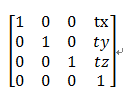旋转矩阵：
X轴旋转矩阵：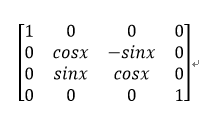Y轴旋转矩阵：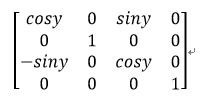Z轴旋转矩阵：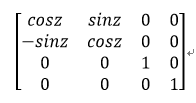缩放矩阵：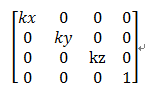变换矩阵，就是将一个向量依次进行 平移、旋转和缩放变换，
设平移矩阵为MatMove，旋转矩阵分别为 MatRotateX、MatRotateY、MatRotateZ，缩放矩阵为MatScale
在Unity中，处理旋转是按照 Z 、 X、Y轴顺序依次进行旋转，所以最终的旋转矩阵
公式一：MatRotate =  MatRotateY x MatRotateX x MatRotateZ
矩阵相乘的顺序要从右往左读，关于旋转矩阵的相乘顺序，还有另一种
公式二：MatRotate =  MatRotateZ x MatRotateX x MatRotateY
两种顺序刚好相反，这是因为关于旋转有两种解读方案，
第一种是，世界坐标系下依次进行Z轴旋转、X轴、Y轴旋转。对应公式一。
第二种是，先在世界坐标系下进行Z轴旋转，同时将世界坐标系一起旋转，得到新的临时坐标系1，
接着在临时坐标系1下进行X轴旋转，同时将临时坐标系1一起旋转，得到新的临时坐标系2，
最后在在临时坐标系2下进行Y轴旋转，得到最后的旋转结果。对应公式二。
Unity中按照第一种顺序相乘。
最终的变换矩阵，就是：
公式三：MatTransfer = MatMove x MatRotate x MatScale
如何用两个已知的向量 反推 变换矩阵，并使用该变换矩阵，将模型所有的顶点变换掉呢？
下面举个例子：
已知模型空间下的向量 A ，求其在世界空间下的向量 A‘
可得：
A' = 变换矩阵 * A
但是如何获得这个 变换矩阵呢？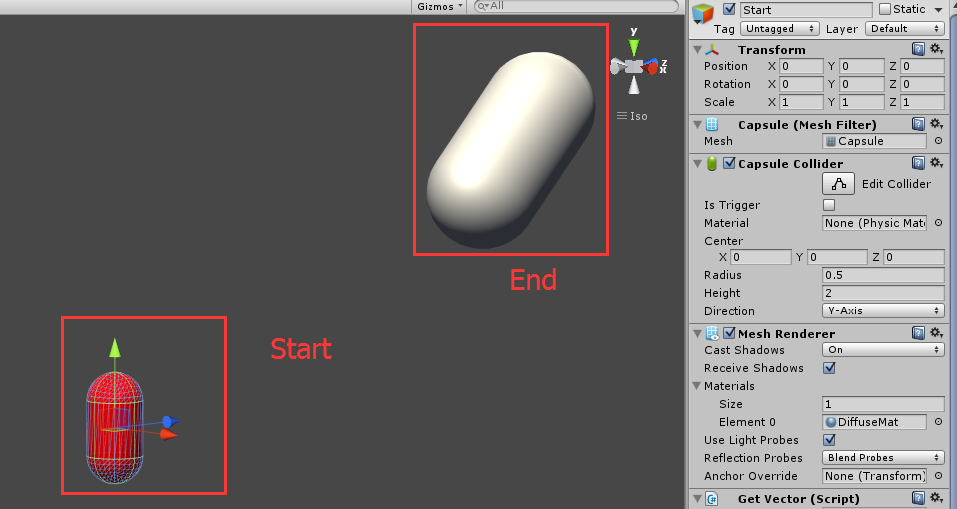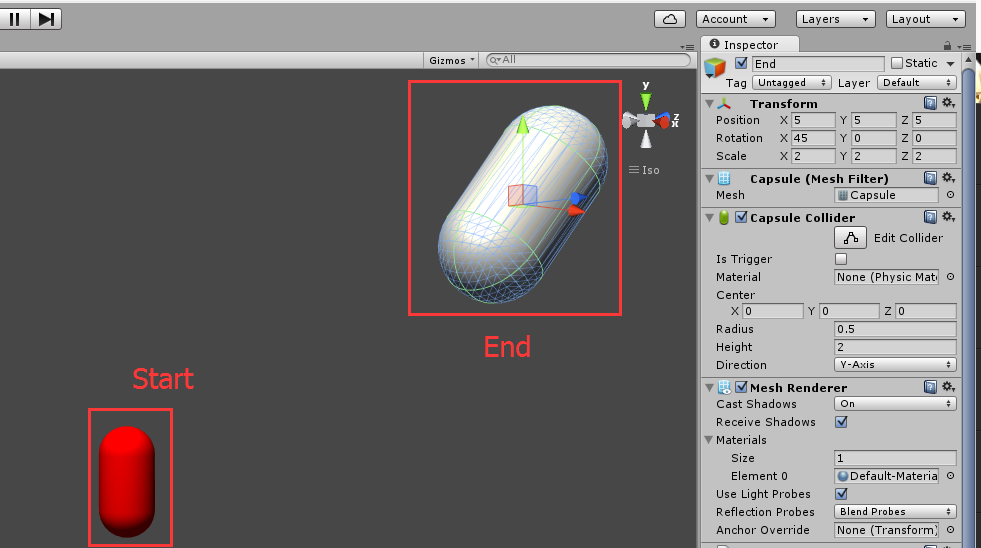如上图，我们想把Start胶囊体最终变换到End胶囊体，在两个胶囊体的Transform组件上获得构建变换矩阵的所有参数。
其中需要两个位置，用来构建平移矩阵。两个向量，用来构建旋转矩阵，分别选为两个胶囊体模型空间下的y轴方向。
还有一个向量，来记录模型沿X、Y、Z方向的缩放。
我们可以用Global和Local切换按钮看到模型空间下的坐标系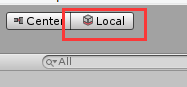模型空间下的坐标系：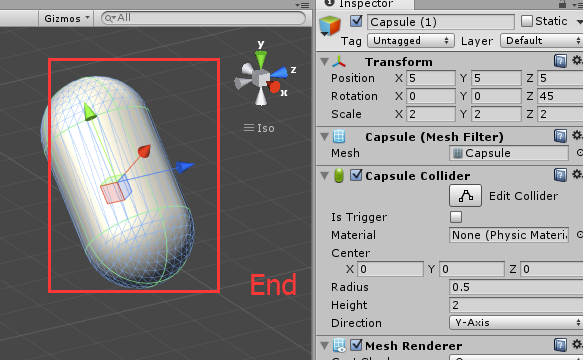我们可以通过访问 transform.up属性直接获得该模型的y轴向量
将下列脚本挂在模型上，右键点击该脚本，会打印出所在模型点的模型空间y轴向量。
（该向量是归一化向量）

using UnityEngine;
using System.Collections;
using UnityEditor;

public class GetVector : MonoBehaviour {

public void GetLocalUp()
{
Debug.Log(name + "的模型空间y轴向量为： " + transform.up.ToString("0.00"));
}
}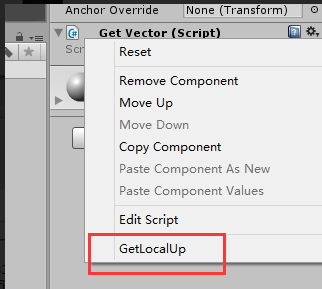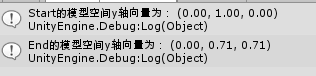接下来开始构建各个变换矩阵

using UnityEngine;
using System.Collections;
using System;
using System.Collections.Generic;

public class TransferMatrix : MonoBehaviour {

public Vector3 StartPos;
public Vector3 EndPos;
public Vector3 StartVec;
public Vector3 EndVec;
public Vector3 Scale;
// Use this for initialization
void Start() {

//平移矩阵
Vector4[] moveTransfer = {
new Vector4(1,0,0,EndPos.x - StartPos.x),
new Vector4(0,1,0,EndPos.y - StartPos.y),
new Vector4(0,0,1,EndPos.z - StartPos.z),
new Vector4(0,0,0,1)
};
Matrix4x4 moveTransferMatrix = new Matrix4x4();
for (int i = 0; i < moveTransfer.Length; i++)
{
moveTransferMatrix.SetRow(i,moveTransfer[i]);
}

//计算两个向量间的欧拉角
Vector3 eular = Quaternion.FromToRotation(StartVec,EndVec ).eulerAngles;
eular.x = Vector3.Angle(Vector3.ProjectOnPlane(StartVec,Vector3.right), Vector3.ProjectOnPlane(EndVec, Vector3.right));
eular.y = Vector3.Angle(Vector3.ProjectOnPlane(StartVec, Vector3.up), Vector3.ProjectOnPlane(EndVec, Vector3.up));
eular.z = Vector3.Angle(Vector3.ProjectOnPlane(StartVec, Vector3.forward), Vector3.ProjectOnPlane(EndVec, Vector3.forward));
//x轴旋转矩阵
float angleX = eular.x == 90f ? 0 : eular.x;
float cosX = (float)Math.Cos((float)Math.PI / 180 * angleX);
float sinX = (float)Math.Sin((float)Math.PI / 180 * angleX);
Vector4[] rotateTransferX = {
new Vector4(1,  0,      0,      0),
new Vector4(0,  cosX,   -sinX,   0),
new Vector4(0,  sinX,   cosX,  0),
new Vector4(0,  0,      0,      1)
};
Matrix4x4 rotateTransferXMatrix = new Matrix4x4();
for (int i = 0; i < rotateTransferX.Length; i++)
{
rotateTransferXMatrix.SetRow(i, rotateTransferX[i]);
}

//y轴旋转矩阵
float angleY = eular.y == 90f ? 0 : eular.y;

float cosY = (float)Math.Cos((float)Math.PI / 180 * angleY);
float sinY = (float)Math.Sin((float)Math.PI / 180 * angleY);
Vector4[] rotateTransferY = {
new Vector4(cosY,  0,   sinY,   0),
new Vector4(0,     1,   0,      0),
new Vector4(-sinY, 0,   cosY,   0),
new Vector4(0,     0,   0,      1)
};
Matrix4x4 rotateTransferYMatrix = new Matrix4x4();
for (int i = 0; i < rotateTransferY.Length; i++)
{
rotateTransferYMatrix.SetRow(i, rotateTransferY[i]);
}

//Z轴旋转矩阵
float angleZ = eular.z == 90f ? 0 : eular.z;

float cosZ = (float)Math.Cos((float)Math.PI / 180 * angleZ);
float sinZ = (float)Math.Sin((float)Math.PI / 180 * angleZ);
Vector4[] rotateTransferZ = {
new Vector4(cosZ,   -sinZ,  0,      0),
new Vector4(sinZ,   cosZ,   0,      0),
new Vector4(0,      0,      1,      0),
new Vector4(0,      0,      0,      1)
};
Matrix4x4 rotateTransferZMatrix = new Matrix4x4();
for (int i = 0; i < rotateTransferZ.Length; i++)
{
rotateTransferZMatrix.SetRow(i, rotateTransferZ[i]);
}

//缩放矩阵
Vector4[] scaleransfer = {
new Vector4(Scale.x,      0,          0,          0),
new Vector4(0,            Scale.y,    0,          0),
new Vector4(0,            0,          Scale.z,    0),
new Vector4(0,            0,          0,          1)
};
Matrix4x4 scaleTransferMatrix = new Matrix4x4();
for (int i = 0; i < scaleransfer.Length; i++)
{
scaleTransferMatrix.SetRow(i, scaleransfer[i]);
}

//Unity中的旋转矩阵：
//Unity中按照  Z、X、Y轴的顺序进行旋转，下式乘法顺序不可变
Matrix4x4 rotateMatrix = rotateTransferYMatrix  * rotateTransferXMatrix  * rotateTransferZMatrix;

//最终变换矩阵
Matrix4x4 transferMatrix = moveTransferMatrix * rotateMatrix *  scaleTransferMatrix;

Matrix4x4 matScale = new Matrix4x4();

matScale.SetTRS(Vector3.zero, Quaternion.Euler(Vector3.zero), new Vector3(Scale.x, Scale.y, Scale.z));
Debug.Log("---------------------------\n");
Debug.Log("测试缩放：\n" + scaleTransferMatrix.ToString("0.00"));
Debug.Log("参照：\n" + matScale.ToString("0.00"));

Matrix4x4 matMove = new Matrix4x4();
matMove.SetTRS(EndPos - StartPos, Quaternion.Euler(Vector3.zero), Vector3.one);
Debug.Log("---------------------------\n");
Debug.Log("测试平移：\n" + moveTransferMatrix.ToString("0.00"));
Debug.Log("参照：\n" + matMove.ToString("0.00"));

Matrix4x4 matRoX = new Matrix4x4();
matRoX.SetTRS(Vector3.zero, Quaternion.AngleAxis(angleX, Vector3.right), Vector3.one);
Debug.Log("---------------------------\n");
Debug.Log("测试旋转矩阵X：\n" + rotateTransferXMatrix.ToString("0.00"));
Debug.Log("参照：\n" + matRoX.ToString("0.00"));

Matrix4x4 matRoY = new Matrix4x4();
matRoY.SetTRS(Vector3.zero, Quaternion.AngleAxis(angleY, Vector3.up), Vector3.one);
Debug.Log("---------------------------\n");
Debug.Log("测试旋转矩阵Y：\n" + rotateTransferYMatrix.ToString("0.00"));
Debug.Log("参照：\n" + matRoY.ToString("0.00"));

Matrix4x4 matRoZ = new Matrix4x4();
matRoZ.SetTRS(Vector3.zero, Quaternion.AngleAxis(angleZ, Vector3.forward), Vector3.one);
Debug.Log("---------------------------\n");
Debug.Log("测试旋转矩阵Z：\n" + rotateTransferZMatrix.ToString("0.00"));
Debug.Log("参照：\n" + matRoZ.ToString("0.00"));

Debug.Log("---------------------------\n");

//利用U3D内置方法算出由向量v1到向量v2的变换矩阵，用来参照
Matrix4x4 referenceMat = new Matrix4x4();
referenceMat.SetTRS(EndPos - StartPos, Quaternion.Euler(eular),new Vector3(Scale.x, Scale.y, Scale.z));

Debug.Log("变换矩阵 : \n" + transferMatrix.ToString("0.00"));
Debug.Log("参照矩阵 : \n" + referenceMat.ToString("0.00"));

List<Vector3> meshList = new List<Vector3>();
Mesh n_mesh = GetComponent<MeshFilter>().mesh;

for (int i = 0; i < n_mesh.vertexCount; i++)
{
}
n_mesh.SetVertices(meshList);
}
}


将脚本挂在Start胶囊体上面，并填写参数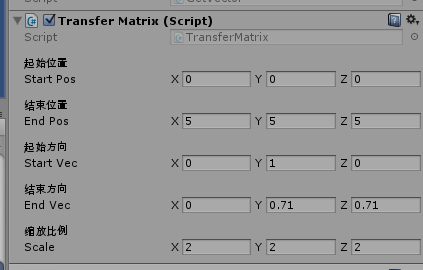运行Unity，可以看到Start胶囊体成功和End胶囊体重合。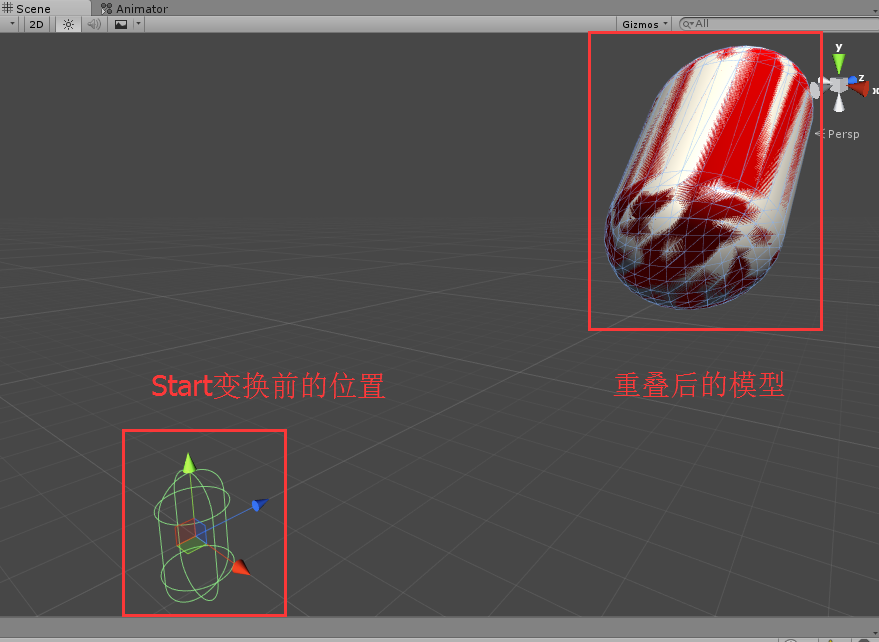在Console中，可以看到各个矩阵的计算结果，作为参照，还使用Unity中自带的构建变换矩阵的方法计算相应的矩阵作为参照， 其中最终的计算矩阵为：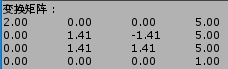展开全文unity 向量
• 为矩阵向量创建 Haar 小波变换矩阵 H Haar小波变换的乘法实现。 此函数使用以下漂亮的公式来创建 Haar 变换矩阵： H_n=1/sqrt(2)[H_(n/2) 克朗 (1 1) I_(n/2) 克朗 (1 -1)], 其中“kron”表示克罗内克积。 迭代从 H_...matlab
• 图像仿射变换原理2：矩阵变换、线性变换和图像线性变换矩阵.pdf
• 【OpenGL】二十、OpenGL 矩阵变换 ( 矩阵缩放变换 | 矩阵旋转变换 | 矩阵平移变换 ) https://hanshuliang.blog.csdn.net/article/details/112859256 博客源码 ( 该源码是 Windows 桌面程序 , 使用 Visual Studio ...OpenGL
• 图像线性变换是仿射变换的子集，包括图像的旋转、错切、缩放以及几者的组合叠加，...所有图像线性变换都可以使用图像变换矩阵和图像平面空间向量的乘积来表示，本文分析了图像线性变换的代数表示方法以及对应变换矩阵
☞ ░ 老猿Python博文目录：https://blog.csdn.net/LaoYuanPython ░
仿射变换博文传送门（带星号的为付费专栏文章）：

*图像仿射变换原理1：齐次坐标来龙去脉详解
*图像仿射变换原理2：矩阵变换、线性变换和图像线性变换矩阵
*图像仿射变换原理3：仿射变换类型及变换矩阵详解
*图像仿射变换原理4：组合变换及对应变换矩阵
*图像仿射变换原理5：组合变换矩阵的OpenCV-Python实现
OpenCV-Py

展开全文图形图像处理
• 音视频资料-图像仿射变换原理2：矩阵变换、线性变换和图像线性变换矩阵.rar
• 讨论了几种线性变换下的矩阵表示，以及线性变换所对应的变换矩阵的形式，在相应变换下给出实例，说明线性变换下的变换矩阵在解决实际问题中应用。
• 本文介绍了组合(也称复合）仿射变换的概念、变换过程以及变换矩阵，并以绕指定点旋转的组合变换、指定直线作为依赖轴的组合变换详细介绍了变换过程和变换矩阵的构成，有助于深入理解仿射变换的概念和处理过程。
☞ ░ 老猿Python博文目录：https://blog.csdn.net/LaoYuanPython ░
仿射变换博文传送门（带星号的为付费专栏文章）：

*图像仿射变换原理1：齐次坐标来龙去脉详解
*图像仿射变换原理2：矩阵变换、线性变换和图像线性变换矩阵
*图像仿射变换原理3：仿射变换类型及变换矩阵详解
*图像仿射变换原理4：组合变换及对应变换矩阵
*图像仿射变换原理5：组合变换矩阵的OpenCV-Python实现
OpenCV-Py

展开全文图形图像处理 仿射变换
• matlab开发-四元数变换矩阵。四元数变换矩阵到欧拉轴
• 根据自定义的单应变换矩阵，对图像进行单应变换，其实不光是单应变换，通过自己设定变换矩阵H，根据xH=x‘，可以进行任意变换，注意变换矩阵不要设定的太离谱
• 本文介绍了仿射变换的类型及其关系以及仿射变换矩阵，基本的仿射变换包括平移、旋转、缩放和错切，镜像可以看做特殊的缩放。实际中一般图像的仿射变换就是平移、旋转、缩放和错切的叠加组合，每叠加一个处理，就进行...图形图像处理 仿射变换 python OpenCV
• 矩阵第四项为齐次坐标,方便几种变换的统一转换 以下任意矩阵可组合,只需及将矩阵相乘得到新的变换矩阵 平移矩阵 旋转矩阵 缩放矩阵
• 文章目录写在前面仿射变换：平移、旋转、放缩、剪切、反射变换矩阵形式变换矩阵的理解与记忆变换矩阵的参数估计参考 写在前面 2D图像常见的坐标变换如下图所示： 这篇文章不包含透视变换（projective/perspective ...
• matlab开发-Haar小波变换矩阵化。创建Haar小波变换矩阵
• 透视变换矩阵： 透视变换矩阵warpMatric，（下图使用的A表示，下面代码该矩阵用warpMatrix表示，A表示8*8的矩阵） 透视变换的源点和目标点，矩阵如下： 源点矩阵： 目标点矩阵： 这是一...python实现
• 解构变换矩阵 给定一个转换的复合矩阵，关于组成该转换的任何单个转换的信息就会丢失。 我们如果有一个复合矩阵，怎么能使其分解为TRS三个矩阵呢？即如何完成下述变化： 其中M是给定的变换矩阵，T是平移矩阵，...
• 1 变换矩阵T的基本形式 T = P为新坐标，P0为原坐标。 T中除了左下角是1以外，其余各个变量都有意义 图像的平面变换又叫仿射变换，与之相对的还有透视变换，不过那就涉及到3维了，这里不提。 2 平移 ....图像的平移 图像的旋转
•SVD
• 1、基本几何变换及变换矩阵 基本几何变换都是相对于坐标原点和坐标轴进行的几何变换，有平移、比例、旋转、反射和错切等。 1.1 平移变换 是指将p点沿直线路径从一个坐标位置移到另一个坐标位置的重定位过程。他...
• 本文简述了一种法向量变换矩阵的推导过程 使用矩阵对点进行空间变换是图形学中的常见操作,假设变换矩阵为 MMM ,我们需要变换切向量 TTT(由 点 P2P_2P2​ - 点 P1P_1P1​ 定义) 以及与其垂直的法向量 NNN .(TTT 和 ...法向量 矩阵 坐标变换
• 已知坐标系 AAA 相对于坐标系 BBB 的齐次变换矩阵为 TABT_{A}^{B}TAB​，有时在求解问题时需要依据 TABT_{A}^{B}TAB​ 求取坐标系 BBB 相对于坐标系 AAA 的齐次变换矩阵 TBAT_{B}^{A}TBA​ ，这个问题可以简单的描述...
• 1. 通过点和角度，获取仿射变换矩阵，进而获取矩阵变换后的图像 Mat dstImage; Mat affine_matrix = getRotationMatrix2D(center, angle, 1.0); warpAffine(img, dstImage, affine_matrix, img.size()); 2. 通过...
• ## 齐次变换矩阵

千次阅读 2019-04-11 21:07:11
• 本文所讲的累积变换矩阵（注:并非几何书上的术语），是指在原有变换矩阵的基础上，乘以一个额外的变换矩阵而得到的新的变换矩阵。 当对图形利用几何变换及其逆变换的时候，特别是利用System.Windows.Media.Matrix...Matrix Geometry Transform...